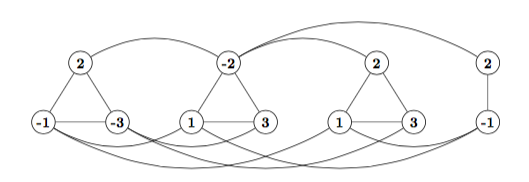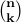# p vs np

history   P   NP   NP-C   sat   21 others   sat->3sat   3sat->IS   clique   wc time

## class P

• decision problem: answer yes or no

• e.g. here is a graph, is there a path between A,B with distance at most 31?

• e.g. here is a graph, does it have a clique of size 32?

• e.g. here is a CNF formula, is it satisfiable?

• class P: set of decision problems that can be solved in polytime

• e.g. at-most-k distance problem: given a graph G, nodes A,B, integer k, is there a path between A,B with distance at most k?

• above usually called shortest path problem, so we say shortest path is in P

• not known:

• k-clique in P ?

• sat (satisfiability) in P ?

## class NP

• decision problem yes(no)-instance: instance of problem where answer is yes(no)

• class NP

• decision problem

• for every yes-instance, exists yes-proof (proof that answer is yes) that can be verified in polytime

• e.g. is sat in NP ?

• yes, because

• consider a yes instance, i.e. a satisfiable formula

• it must have a satisfying assignment

• so a yes-proof for sat is a satisfying assignment

• if someone gives you a formula in CNF, and an assignment, how long does it take you to verify that this assignment satisfies the formula?

• you assign truth values to the n variables, time O(n)

• for each clause, you check that the assignment is satisfied, time O(nm)

• so in O(nm) time you can verify that the formula is satisfiable

• so sat is in NP

## class NP-C

• class NP-C: NP-complete problems

• informally, each problem in NP-C is as hard as any problem in NP

• formally, a problem X in NP is complete if, for each problem A in NP, there is a polytime process that

• takes an instance a of A

• converts it into an instance x of X

• preserves yes-answers: answer(a) is yes if and only if answer(x) is yes

• consequence

• suppose exists polytime algorithm for some such X

• then any problem in NP can be solved in polytime

• above consequence is why we say NP-C are hardest problems in NP

## 21 others NP-C

• suppose we want to show problem X is NP-complete (so, hard)

• what can we do ?

• 1) show X is in NP

• 2) show that some NP-complete problem C can be poly-transformed into X, so that answers (yes/no) are same for C instance and transformed X instance

• 3) then we have shown X is NP-complete. why ?

• because any problem T in NP can be transformed into sat, and then into X

• so if we could solve X in poly T, we could solve T in poly time

• 1972 (Karp) 21 problems NP-C:

• 3-sat (each clause has ≤ 3 literals)

• clique

• knapsack

• set cover

• ...

• Karp's 21 reductions

## sat reduces to 3-sat

example reduction
```consider an instance of sat:
[  1  2  3  -4  -6 ]
[  1  2 -3  -5     ]
[  1 -2  3  -5  -6 ]
[  1  3  4         ]
[  2 -3  4 -6      ]

replace each long clause with
equivalent set of 3-literal clauses
[ 1  2  3 -4 -6]  => [ 1 2 10] [-10 3 11] [-11 -4 -6]
[ 1  2 -3 -5]     => [ 1 2 12] [-12 -3 -5]
[ 1 -2  3 -5 -6]  => [ 1 -2 13] [-13 3 14] [-14 -5 -6]
[ 2 -3  4 -6]     => [ 2 -3 15] [-15 4 -6]

corresponding instance of 3-sat
[   1   2  10 ]
[ -10   3  11 ]
[ -11  -4  -6 ]
[   1   2  12 ]
[ -12  -3  -5 ]
[  1   -2  13 ]
[ -13   3  14 ]
[ -14  -5  -6 ]
[   1   3   4 ]
[   2  -3  15 ]
[ -15   4  -6 ]
```
above reduction implies 3-sat is NP-C
• number literals in new instance < 3 times original, so reduction polytime

• reduction maps yes-instance to yes-intance, no-instance to no-instance

• by Cook's theorem

• sat is NP-C

• so any instance t of problem T in NP can be polytime reduced to instance s of sat, with answer preserved

• as above, instance s can be polytime reduced to instance u of 3-sat, with answer preserved

• so t can be polytime reduced to u, with answer preserved

• so polysolving 3-sat implies polysolving T, for every T in NP

• so 3-sat is NP-complete

clique and independent set
• clique in a graph, set of nodes, each pair adjacent

• independent set in a graph, set of nodes, each pair non-adjacent

• independent set decision problem: given a graph G and an integer k, does G have an independent set of size k ?

• clique decision problem: given a graph G and an integer k, does G have a clique of size k ?

## 3-sat reduces to ind. set

example reduction
• consider an instance of 3-sat

• each clause with t literals becomes clique with t nodes

• e.g. clause [ a b -c ] becomes clique {a b -c}

• for each literal pair x,-x from different clauses, add edge in graph

• e.g. what is graph reduced from this CNF formula?

[-1 2 -3] [ 1 -2 3] [ 1 2 3] [-1 2]claim: formula satisfiable iff graph has m-independent set
• assume formula satisfiable, say with assignment A

• in each clause, at least one literal true

• in graph, pick one true node from each clause

• picked nodes form independent set (why?)

• so graph has m-ind set

• assume graph has m-ind set

• set has exactly one node in each clauseclique

• set corresponding literal true

• cannot have both x and -x true (why?)

• each clause satisfied by this assignment

consequence of claim ?
• independent set is NP-C :)

• why ?

exercise

show independent set reduces to clique

consequence of exercise ?
• clique is NP-C :)

• why ?

## brute force k-clique time

• try each k-subset

• (n choose k) == n! / (k!) (n-k)! = n(n-1)...(n-(k-1)) / k! subsets

• checking each takes Θ(k2) time

• if k constant, Θ(nk) subsets, total WC time Θ(k2 nk) = Θ(nk)   polynomial :)

• here, WC time polynomial :)

• if k not constant, e.g. can grow with n ?

• largest (n choose k) ? when k = n/2 (CMPUT 272)

• how big is (n choose n/2) ?

•   n!   ≈   √  2 π n  (n/e)n   (Stirling)

•   n!   ≈   above * e1/12   (better)

• (n choose n/2)   =   n!   /   ( (n/2)!   (n/2)! )

• ... school algebra : substitute Stirling approx ...

•   ≈   2n /  √   π n / 2

•   ≈   above * e-1/4   (better)

• try each subset, worst case runtime Θ(   n1.5 2n   )

• here, WC time exponential :(

1000 choose 500
```2.7035582144 e+299   Stirling approx
2.7028824093 e+299   better     "
2.7028824095 e+299   exact (rounded)
```

## wc time for some NP-C problems

• brute force clique, also independent set

• Θ(   n1.5 2n   )

• knapsack, n items, each value/wt n bits, capacity .5 total wt, input size t = Θ( n2 )

• Θ( n2 2n )   =   Θ( t 2√ t )

• brute force set cover, m subsets of an n-set

• Θ( n 2m )

• brute force sat, total m literals, n variables

• Θ( m 2n )